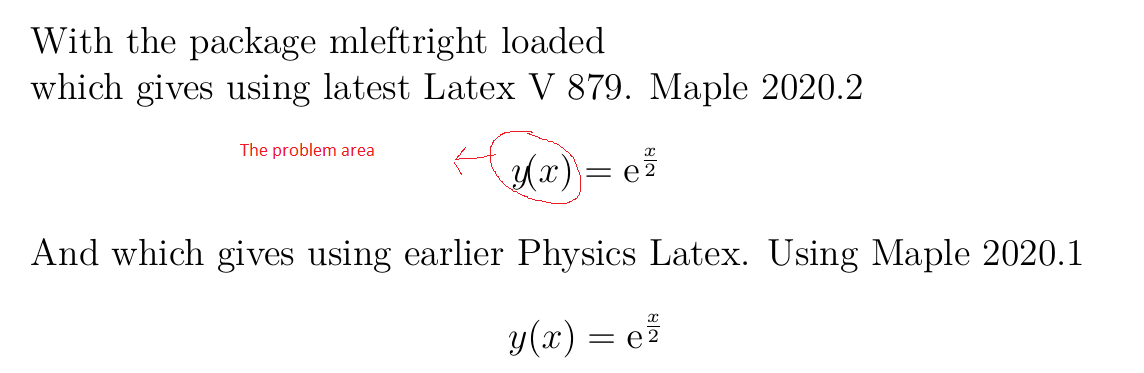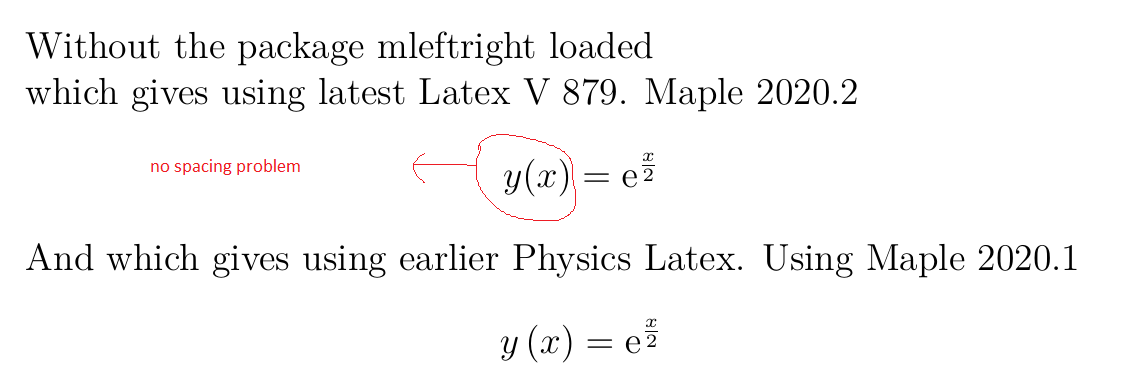:

## small note about the new Maple 2020.2 and Latex V.879 and mleftright package

Maple 2020

I am testing Maple 2020.2 with new Latex with Physics version latest 879.

The latex generated now issues \! between the symbol and the () next to it to improve the spacing. This post is just to let anyone using the package mleftright in Latex, that this will cause a problem. So it is better to remove this package if you are allready using it.

Here is an example

eq:=y(x)=exp(x/2);
Latex(eq)

y \! \left(x \right) = {\mathrm e}^{\frac{x}{2}}

In earlier version of Physics:-Latex (now it is just Latex), the above generated this

             y  \left(x \right) = {\mathrm e}^{\frac{x}{2}}

Notice, no \! in earlier version.

If you happen to be using \usepackage{mleftright} to improve the spacing for \left and \right, which I was using, you'll get negative side effect. A solution is to remove this package. Here is an example showing the above Latex compiled with this package added, and without it, so you can see the differerence.

\documentclass[12pt]{book}
\usepackage{amsmath}
\usepackage{mleftright}
\mleftright
\begin{document}

which gives using latest Latex V 879. Maple 2020.2
$y \! \left(x \right) = {\mathrm e}^{\frac{x}{2}}$

And which gives using earlier Physics Latex. Using Maple 2020.1
$y \left(x \right) = {\mathrm e}^{\frac{x}{2}}$
\end{document}This is the output without using this package. by removing the inlcude command in the above Latex code and not calling mlfright. Now the problem is gone:I like the effect added from \! , which is a manual way to improve the space, which this package was doing.

just be careful not to use mleftright package now, which is a somewhat popular package in latex and It was recommended to use sometime ago to improve the spacing, as it will over correct the spacing, and looks like not needed any more with latest Maple Latex.﻿